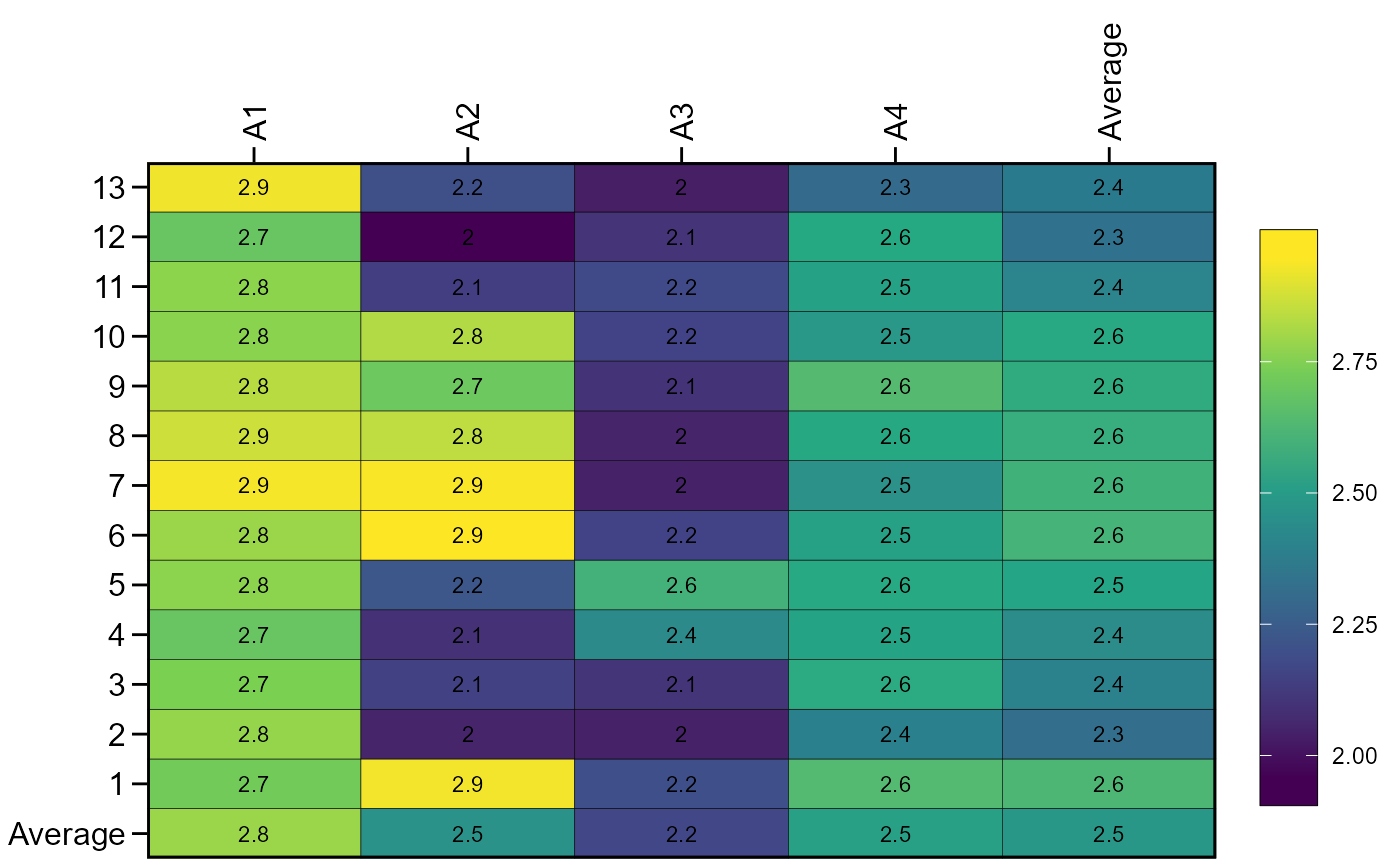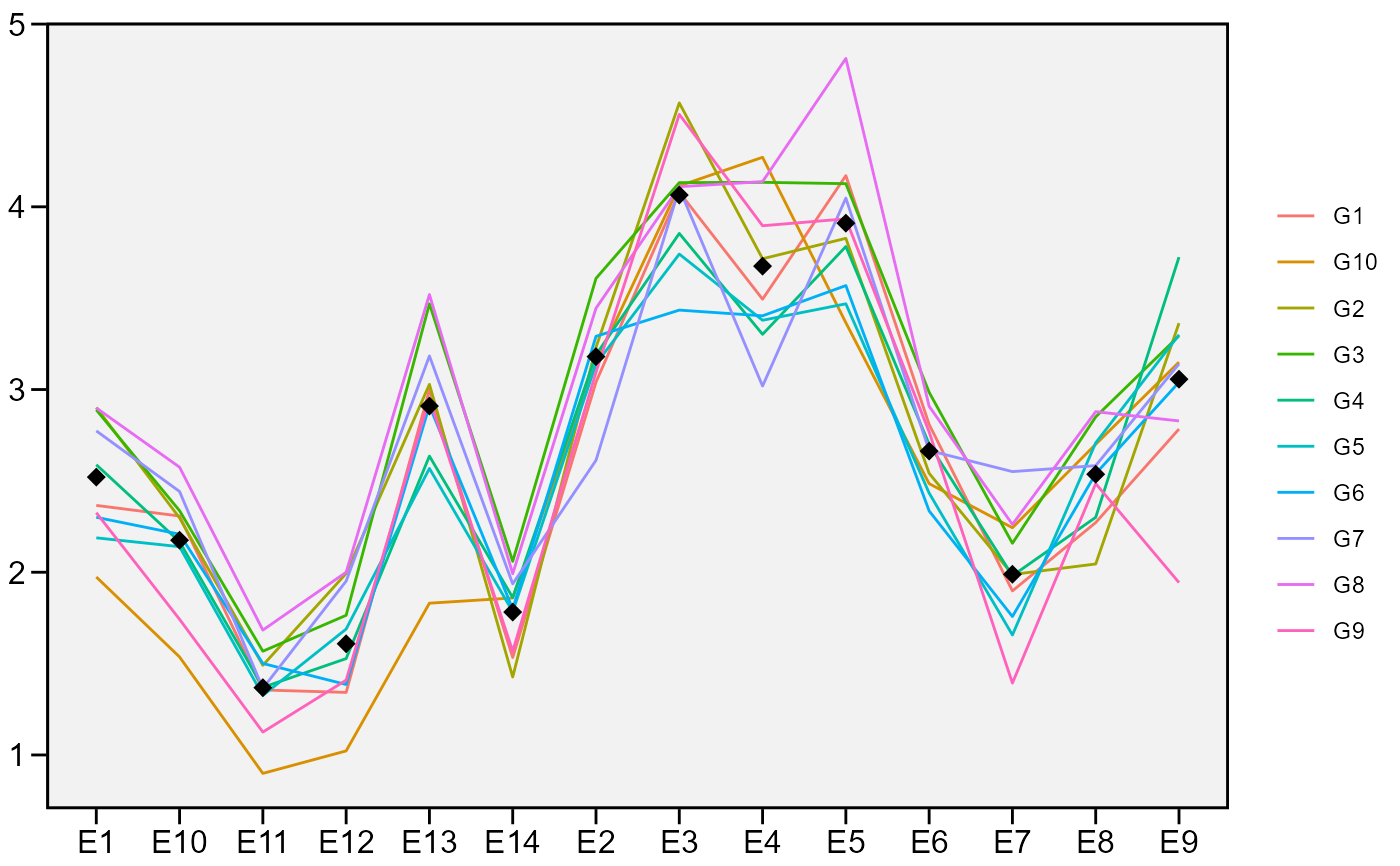This function produces a line plot for a graphical interpretation of the genotype-vs-environment interaction. By default, environments are in the x axis whereas the genotypes are depicted by different lines. The y axis contains the value of the selected variable. A heatmap can also be created.

## Usage

ge_plot(
.data,
env,
gen,
resp,
type = 1,
values = TRUE,
text_col_pos = c("top", "bottom"),
text_row_pos = c("left", "right"),
average = TRUE,
order_g = NULL,
order_e = NULL,
xlab = NULL,
ylab = NULL,
width_bar = 1.5,
heigth_bar = 15,
plot_theme = theme_metan(),
colour = TRUE
)

## Arguments

.data

The dataset containing the columns related to Environments, Genotypes, replication/block and response variable(s).

env

The name of the column that contains the levels of the environments

gen

The name of the column that contains the levels of the genotypes.

resp

The response variable.

type

The type of plot type = 1 for a heatmap or type = 2 for a line plot.

values

Show the values in the plot? Defaults to TRUE.

text_row_pos, text_col_pos

The position of the text in the rows and columns. The defaults show the text at left and top.

average

Show the average values for environments and genotypes? Defaults to TRUE.

order_g, order_e

A charactere vector indicating the order of the levels for genotypes and environments, respectively. This can be used to change the default ordering of rows and columns.

xlab, ylab

The labels for x and y axis, respectively.

width_bar, heigth_bar

The width and heigth of the legend bar, respectively.

plot_theme

The graphical theme of the plot. Default is plot_theme = theme_metan(). For more details,see ggplot2::theme().

colour

Logical argument. If FALSE then the plot will not be colored.

## Value

An object of class gg, ggplot.

## Author

Tiago Olivoto tiagoolivoto@gmail.com

## Examples

# \donttest{
library(metan)
ge_plot(data_ge2, ENV, GEN, PH)ge_plot(data_ge, ENV, GEN, GY, type = 2)# }